Overview

This guide is an introduction to the Rsampling package, which replicates in R the functions of the Resampling Stats add-in for Excel. (http://www.resample.com/) 1.

These functions are used in a workflow that summarizes the logic behind significance tests:

1. Define a statistic of interest;
2. Define the null hypothesis;
3. Get the statistic of interest distribution under null hypothesis;
4. If the probability of the observed statistic of interest occurring under null hypothesis is lower than a critical value, reject the null hypothesis.

Resampling Stats’s main idea is to facilitate the understanding of this logic, by making the user execute each step in a spreadsheet, with the aid of some macros.

Rsampling’s package aims at enabling this same training process in R. Keeping the original workflow is favored over performance.

The sections following installation instructions are examples of the simpler and most common applications of Rsampling. You may refer to the package help pages to learn about all other functionalities.

Installation

Rsampling is hosted on CRAN. To install it use

> install.packages("Rsampling")

You can also install from the GitHub site, where the project is hosted. To do that use the devtools package function install_github:

> library(devtools)
> install_github(repo = 'lageIBUSP/Rsampling')

> library(Rsampling)

Shuffling a column to test difference between groups

The embauba data frame contains the data on presence and absence of lianas on Cecropia trees of two morphotypes (white and red).

morphotype with.vines
1   VERMELHA      FALSE
2   VERMELHA      FALSE
3   VERMELHA      FALSE
4   VERMELHA      FALSE
5   VERMELHA      FALSE
6   VERMELHA      FALSE
> summary(embauba)
morphotype with.vines
BRANCA  :82   Mode :logical
VERMELHA:70   FALSE:105
TRUE :47
NA's :0

For more details on the data please refer to help page (?embauba).

Study hypothesis

The original study’s hypothesis is that ants that live within the hollow trunks of Cecropia remove lianas from these trees. The prediction is that Cecropia trees of the red morphotype would be less infested by lianas than the white ones, because they shelter ant colonies more often. In fact, this difference is observed in the proportions of trees infested by lianas in the sample:

> tapply(embauba$with.vines, embauba$morphotype, mean)
BRANCA  VERMELHA
0.4878049 0.1000000

Null hypothesis

The null hypothesis is that the proportion of infested trees are equal at the population from where the samples were drawn. Under this hypothesis, a liana has the same chance of occurrence in both morphotypes. We can simulate this null hypothesis by shuffling the presence of lianas between trees in the data table.

Statistic of interest

For each simulation we have to calculate our statistic of interest, which is the difference of infestation by lianas between the two morphotypes. So, we create a function to calculate this statistic:

> emb.si <- function(dataframe){
+     props <- tapply(dataframe$with.vines, dataframe$morphotype, mean)
+     props[] - props[]
+ }
> ## Verifying
> emb.si(embauba)
 0.3878049

Distribution of statistics under the null hypothesis

Then we run the simulation with the function Rsampling:

> emb.r <- Rsampling(type = "normal", dataframe = embauba,
+                    statistics = emb.si, cols = 2, ntrials = 1000)

What does this command mean?

• type = "normal" indicates a randomization of a whole data set (the most basic type of randomization, afterwards you’ll see other types).
• dataframe = embauba indicates the data table
• statistics = emb.si indicates the function that calculates the statistic of interest from the data table.
• cols = 2 indicates that the randomization must be done over the second column of the data table.
• ntrials = 1000 indicates the number of simulation repetitions.

The distribution of the statistic of interest at the simulation didn’t even include the observed value:

> dplot(emb.r, svalue = emb.si(embauba), pside="Greater",
+       main = "Frequency distribution of the statistic of interest under H0",
+       xlab = "Statistic of interest")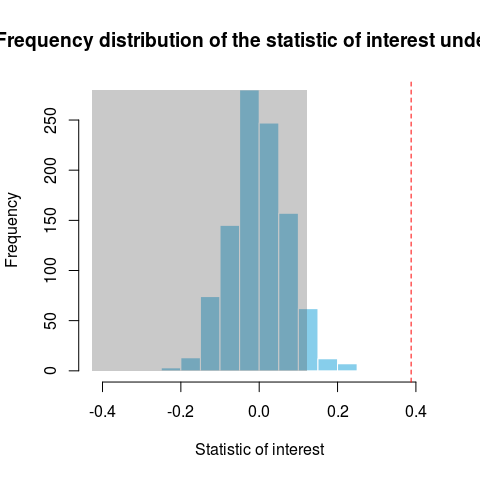Distribution of the differences between the proportions of red and white Cecropia trees with lianas obtained from 1000 simulations of the null hypothesis. The null hypothesis is that the proportion of infested trees are equal at the population from where the samples were drawn. The red line marks the difference observed in the samples and the gray area is the acceptance region of the null hypothesis.

Decision: should we reject the null hypothesis?

As usual in the biological sciences, we adopt the criteria of rejecting the null hypothesis if the probability of the statistic of interest under the null hypothesis is under 5% (p < 0.05).

The area not highlighted in gray marks the top 5% of the statistic distribution under the null hypothesis. Thus, if the observed statistic is in the gray area we do not reject the null hypothesis. This is called the acceptance region of H0. As the observed value (red line) is outside the acceptance region, H0 can be rejected. You can also check this with a logical test in R:

> sum(emb.r >= emb.si(embauba))/1000 < 0.05
 TRUE

Conclusion: we reject the null hypothesis (p < 0.05).

Shuffling within rows to test differences within pairs

The dataframe azteca contains the number of Azteca sp ants recruited by aqueous extracts of old and young leaves of Cecropia trees.

plant extract.new extract.old
1     1           5           0
2     2          12           6
3     3          30           5
4     4           0           0
5     5           0           2
6     6           5           2
> summary(azteca)
plant     extract.new     extract.old
Min.   : 1   Min.   : 0.00   Min.   : 0.000
1st Qu.: 6   1st Qu.: 2.00   1st Qu.: 1.000
Median :11   Median : 4.00   Median : 2.000
Mean   :11   Mean   :10.05   Mean   : 2.714
3rd Qu.:16   3rd Qu.:12.00   3rd Qu.: 4.000
Max.   :21   Max.   :50.00   Max.   :10.000

Study hypothesis

The study hypothesis is that recruitment is more intense when a young leaf is damaged. This hypothesis predicts that an extract made from young leaves would recruit more ants than an extract made of old leaves. Indeed this was the outcome of the experiment:

> splot(azteca$extract.new, azteca$extract.old,
+            groups.names=c("Young leaves","Old leaves"),
+            ylab="Number of recruited ants",
+            xlab="Extract type")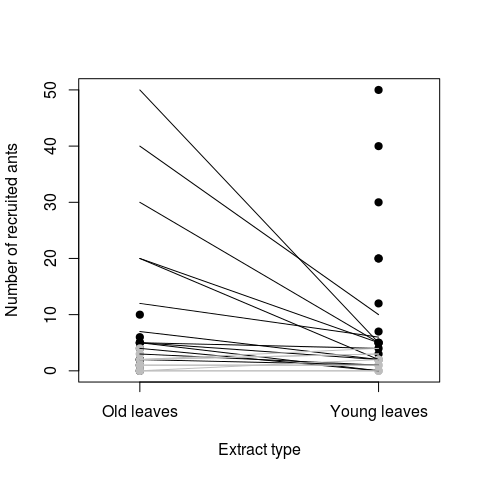Number ants that were recruited by aqueous extracts of young and old leaves of Cecropia trees. The extracts were dropped in paired leaves of Cecropia trees that had colonies of Azteca ants. The lines link leaves of same experimental pair.

Null hypothesis

The null hypothesis is that the recruitment caused by each extract is the same. Note that the experiment was paired in order to control for the other sources of variation. Thus, to simulate the null hypothesis we have to shuffle the number of recruited ants within each pair of leaves.

Statistic of interest

For each simulation we calculate our statistic of interest, which is the mean of the difference of recruited ants within each pair of leaves. We thus create a function calculate the statistic of interest from the data table:

> azt.si <- function(dataframe){
+     diferencas <- with(dataframe, extract.new - extract.old)
+     mean(diferencas)
+ }
> ## Observed value
> azt.si(azteca)
 7.333333

In the experiment the young leaf extract recruited on average 7.3 ants than the old leaf extract, for each pair.

Distribution of the statistic under null hypothesis

As the pairs are at rows of our dataframe, we simulate the null hypothesis shuffling values within each row:

> azt.r <- Rsampling(type = "within_rows", dataframe = azteca,
+                    statistics = azt.si, cols = 2:3, ntrials = 1000)

We changed the argument type = "within rows" to indicate that the values are now shuffled within the lines. The argument cols = 2:3 indicates the columns of the dataframe which contain the counts to be shuffled.

A difference equal to or greater than that observed was very rare in the distribution of the statistic of interest:

> dplot(azt.r, svalue = azt.si(azteca), pside="Greater",
+       main = "Distribution of the statistic of interest under H0",
+       xlab = "Statistic of interest")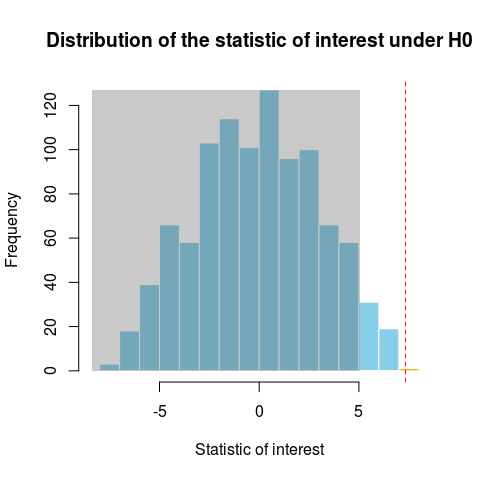Frequency distributions of the difference of the number of ants recruited by the two types of extracts in 1000 simulations of the null hypothesis. The null hypothesis is that both extracts recruit ants equally. The red line marks the difference observed in the samples and the gray area is the acceptance region of the null hypothesis.

Decision: should we reject the null hypothesis?

Again, the distribution of the statistic of interest shows that the observed value of the statistic is outside the acceptance region for the null hypothesis under our significance criterion (5% chance of error). The same result is found with the following logical test:

> sum(azt.r >= azt.si(azteca))/1000 < 0.05
 TRUE

Conclusion: we reject the null hypothesis (p<0.05).

Coda: one-sided and double-tailed tests.

So far we have tested the hypothesis that a value equal to or higher than the observed can be generated by the null hypothesis. We call this an one-tailed or one-sided test, as it would also be if our aim was testing if an equal or smaller value could be generated under null hypothesis. In one-sided tests, the acceptance region comprises the null distribution except its 5% more extreme values.

But we might investigate differences among groups without specifying its direction. For example, prior knowledge could suggest the hypothesis that extracts of young and old leaves should recruit different numbers of ants, but without any expectation concerning which extract would recruit more. This is a case for a two-tailed or two-sided test. In a two-tailed test the acceptance region is the center of the null distribution, excluding their 2.5% most extreme values at each side:

> dplot(azt.r, svalue = azt.si(azteca), pside="Two sided",
+       main = "Two-tailed test",
+       xlab = "Statistics of interest")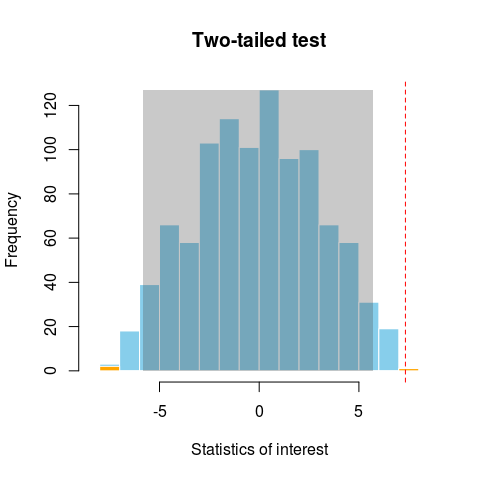Frequency distributions of the difference of the number of ants recruited by the two types of extracts in 1000 simulations of the null hypothesis. The null hypothesis is that both extracts recruit ants equally. The red line marks the difference observed in the samples and the gray area is the acceptance region of the null hypothesis for a two-tailed test

Randomization with replacement

The data frame peucetia contains data from an experiment of substrate choice by spiders of the genus Peucetia. Twenty-seven spiders were kept in Petri dishes covered with two substrata (plant leaves with and without glandular “hairs” - trichomes - ). Every plate was inspected six times to check if each spider was on the leaves with or without trichomes.

t1    t2    t3    t4    t5    t6
1 FALSE FALSE FALSE FALSE FALSE FALSE
2 FALSE FALSE FALSE FALSE FALSE FALSE
3 FALSE FALSE FALSE FALSE FALSE FALSE
4  TRUE  TRUE FALSE FALSE FALSE FALSE
5  TRUE  TRUE  TRUE FALSE FALSE FALSE
6 FALSE FALSE FALSE  TRUE  TRUE  TRUE

Study hypothesis

The study hypothesis is that spiders prefer to hunt in plants with glandular hairs, where catching prey is easier. The predicted outcome of the experiment is that spiders should be recorded most of the time on leaves with trichomes. In fact, most of the spiders were on the leaves with trichomes in at least 4 out of six inspections:

> ## Number of records in leaves with trichomes
> n.insp <- apply(peucetia, 1, sum)
> barplot(table(factor(n.insp, levels=0:6)),
+         xlab="Number of records in leaves with trichomes",
+         ylab="Number of spiders")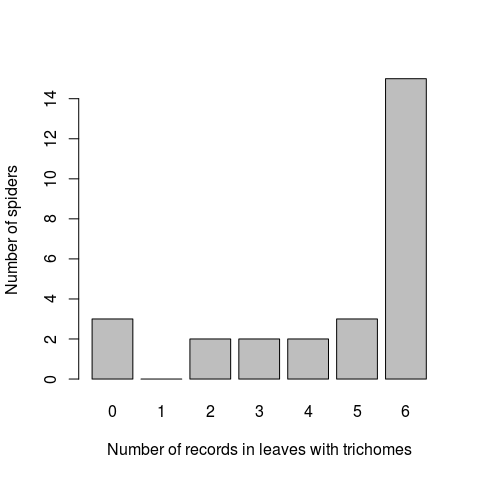Number of inspections each of 27 spiders were recorded on leaves with trichomes in a experiment of preference for two types of substrata (leaves with or without trichomes). The substrate of each spider was checked six times.

Null hypothesis

The null hypothesis is that there is no preference for any substrate. Half of the area of each plate was covered with each leaf type. Hence the null expectation is that the spiders would be in the area covered by leaves with trichomes in half of the inspections. This expectation assumes that each inspection is an independent event.

Statistics of interest

For each simulation we have to calculate our statistic of interest. In this case we choose the average number of inspections where the spiders were recorded on leaves with trichomes. Thus we build a function to calculate this statistics from data:

> peu.si <- function(dataframe){
+     mean(apply(dataframe, 1, sum))
+ }
> ## Observed value
> peu.si(peucetia)
 4.555556

The spiders were recorded on average 4.56 of the six inspections on the area covered with leaves with trichomes.

Distribution of the statistic under the null hypothesis

To simulate our null hypothesis, we create a data frame with the same structure, wherein each spider is on leaves with trichomes on half of the inspections.

> peu.H0 <- matrix( rep(c(TRUE,FALSE), each = 3),
+                  nrow = nrow(peucetia), ncol = ncol(peucetia), byrow=TRUE)
> ## Converts in data.frame
> peu.H0 <- data.frame(peu.H0)
> ## verifying
X1   X2   X3    X4    X5    X6
1 TRUE TRUE TRUE FALSE FALSE FALSE
2 TRUE TRUE TRUE FALSE FALSE FALSE
3 TRUE TRUE TRUE FALSE FALSE FALSE
4 TRUE TRUE TRUE FALSE FALSE FALSE
5 TRUE TRUE TRUE FALSE FALSE FALSE
6 TRUE TRUE TRUE FALSE FALSE FALSE

Then we simulate the null hypothesis by sampling each line with replacement 2:

> peu.r <- Rsampling(type = "within_rows", dataframe = peu.H0,
+                    statistics = peu.si, ntrials = 1000, replace=TRUE)

The argument replace = TRUE indicates sampling with replacement. In this case, this is a drawn of an independent position for each spider at each inspection. Therefore the probability of each spider being at the area covered by leaves with trichomes is 0.5 per drawing.

An average number of records in leaves with trichomes equal to or greater than that observed did not occur at the simulated distribution of our statistic of interest:

> dplot(peu.r, svalue = peu.si(peucetia), pside="Greater",
+       main = "Frequency distribution of the statistics of interest under H0",
+       xlab = "Statistics of interest")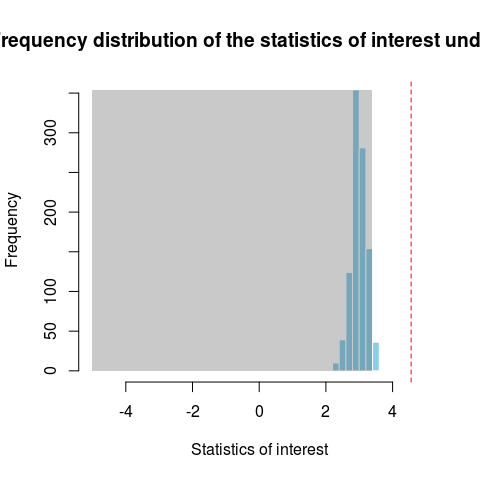Frequency distribution of the mean number of records of spiders on leaves with trichomes in 1000 simulations of the null hypothesis that there is no preference for substrata. The red line marks the observed average in the experiment and the gray area is the acceptance region of the null hypothesis.

Decision: should we reject the null hypothesis?

Again we have a one-tailed test, and the observed value of the statistic of interest fell outside the region of acceptance of the null hypothesis (5%). We can check this with the logical test of our significance criterion:

> sum(peu.r >= peu.si(peucetia))/1000 < 0.05
 TRUE

Conclusion: we reject the null hypothesis (p < 0.05).

A more realistic null hypothesis?

In the previous example we simulated the null hypothesis by drawing a substrate type for each spider for every inspection. In doing that we assumed that the substrate where a spider is in an inspection does not affect where the spider will be in the next inspections. In other words, we assumed that the inspections are independent events.

But what if the records correlate among inspections? This can happen if spiders move at a smaller frequency than the interval between inspections. If this is true, subsequent records for a leaf type may indicate only that spiders tend to stay put, instead of preference. In this case the null hypothesis should keep the number of inspections for each leaf type, altering only the substrata types.

Null hypothesis

The proportion of inspections that spiders remain in one of the substrata does not depend on the substrate type (leaves with or without trichomes).

Therefore the null hypothesis is about the independence between the number of inspections and type of substrate. We simulate this scenario by scrambling the number of occasions between substrata, for each spider. For this we will create a data frame with the observed the number of records of each spider in each substrate:

> ## N of records in leaves with trichomes
> tric <- apply(peucetia, 1, sum)
> ## N of records in leaves w/o trichomes
> lisa <- apply(peucetia, 1, function(x) sum(x==0))
> ## Assembles a data frame with the vectors above
> peu.H0b <- data.frame(tric=tric, lisa = lisa)
> ## Checking 1st lines
tric lisa
1    0    6
2    0    6
3    0    6
4    2    4
5    3    3
6    3    3

Statistic of interest

A statistic of interest can be applied to test different null hypotheses. So we keep the same from our previous example: the average number of inspections where spiders were recorded on leaves with trichomes.

But since the data frame to be randomized has changed, we create a new function in R to calculate the statistic of interest

> peu.si2 <- function(dataframe) mean(dataframe$tric) > ## Checking, should be the same a previous calculation > peu.si2(peu.H0b)  4.555556 Distribution of the statistic of interest under the null hypothesis In this new case, the null hypothesis is simulated by shuffling the rows of the data frame: > peu.r2 <- Rsampling(type = "within_rows", dataframe = peu.H0b, + statistics = peu.si2, ntrials = 1000) The null distribution changed significantly when compared to the previous section. But an average equal to or greater than the observed remained very rare: > dplot(peu.r, svalue = peu.si(peucetia), pside="Greater")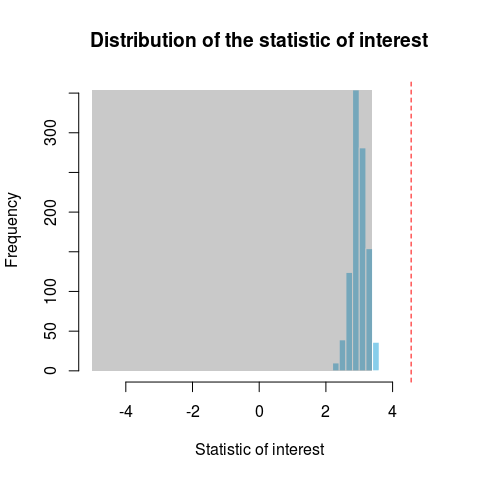Frequency distribution of the mean number of records of spiders on leaves with trichomes in 1000 simulations of the null hypothesis that there is no preference for substrata, but taking into account the tendency of spiders to stay put between inspections. The red line marks the observed average in the experiment and the gray area is the acceptance region of the null hypothesis. > dplot(peu.r2, svalue = peu.si2(peu.H0b), pside="Greater", + main = "Frequency distribution of the statistic of interest under H0", + xlab = "Statistic of interest")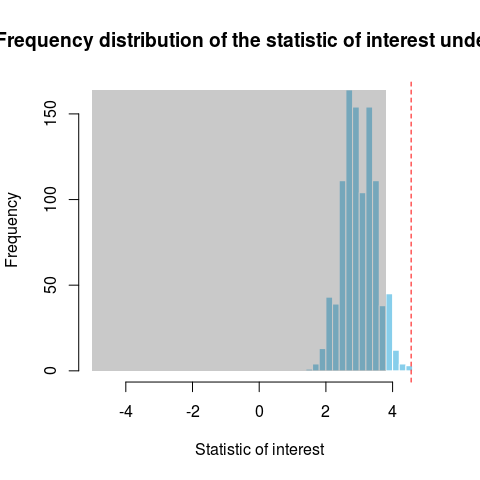Frequency distribution of the mean number of records of spiders on leaves with trichomes in 1000 simulations of the null hypothesis that there is no preference for substrata, but taking into account the tendency of spiders to stay put between inspections. The red line marks the observed average in the experiment and the gray area is the acceptance region of the null hypothesis. Decision: do we reject the null hypothesis? The observed value of the statistic of interest is not within our acceptance region. Applying our significance criterion: > sum(peu.r2 >= peu.si(peucetia))/1000 < 0.05  TRUE Conclusion: we reject the null hypothesis (p < 0.05). Structural zeros Some data sets have observations with zero frequency that are considered impossible to occur or be observed. Obvious cases are a cross table of gender and diseases where records of women with prostate cancer is zero. A less obvious example is in the dataframe pielou, which has the number of records of ten aphid species in twelve species of plants of the genus Solidago sites across Canada. > pielou canadensis nemoralis rugosa juncea gigantea hispida nigrotuberculatus 55 11 19 13 5 1 nigrotibius 9 39 4 5 3 3 caligatus 24 6 22 1 4 0 pieloui 8 1 11 9 8 9 canadensis 8 5 2 5 0 4 gravicornis 8 4 2 5 4 0 lanceolatus 3 2 3 1 1 0 macgillivrayae 0 1 0 1 0 0 erigeronensis 2 0 0 0 0 0 solirostratus 0 0 0 0 0 0 squarrosa graminifolia caesia uliginosa flexicaulis nigrotuberculatus 2 1 1 0 0 nigrotibius 0 0 2 4 1 caligatus 0 1 0 0 0 pieloui 4 4 1 1 0 canadensis 2 0 0 0 0 gravicornis 1 0 0 0 0 lanceolatus 0 0 1 0 0 macgillivrayae 0 2 0 0 0 erigeronensis 0 1 0 0 0 solirostratus 0 0 0 0 1 ohioensis nigrotuberculatus 0 nigrotibius 0 caligatus 0 pieloui 0 canadensis 0 gravicornis 0 lanceolatus 1 macgillivrayae 0 erigeronensis 0 solirostratus 0 To learn more about this data set refer to the help page (?pielou). There are several instances with zero frequency. We’ll simulate a null hypothesis assuming these frequencies are structural, that is, assuming that zeros indicates insect-plant associations that can not occur. This can be a reasonable assumption for phytophagous insects, that are in general highly specialized in some host plants. Study Hypothesis Our research hypothesis is that there is or there was resource partitioning of resources among aphid species. In this case, the observed associations should have resulted in decreased insect niche overlap. Null hypothesis Our null hypothesis is that the niche overlap does not differ from the expected if the use plants by plants are independent. Statistics of interest This data was used to illustrate a method to calculate niche breadth and niche overlap. The expression proposed by the author for the average niche overlap is the difference between the Brillouin index of all values and the sum of columns of the data table. The Brillouin index is a diversity measure in a collection of values$ x_i \$:

$H = \frac{1}{N} \log N! \ - \ \frac{1}{N} \sum \log x_i !$

Where $$N = \sum x_i$$. Let’s then create a function to calculate this index:

> brillouin <- function(x, base=10) {
+     N <- sum(x)
+     lfactorial(N)/(log(base)*N)  -  sum(lfactorial(x)/log(base))/N
+ }

Then we create a function to calculate our statistic of interest

> pielou.si <- function(dataframe)
+     brillouin( dataframe ) - brillouin( apply(dataframe,2,sum) )

And apply the function to the aphid data:

> pielou.si(pielou)
 0.5804034

Distribution of the statistic of interest under the null hypothesis

To simulate our null hypothesis, we shuffle the numbers of records of each species of aphids among the plants. Thus we simulate a situation where we kept the observed aggregation of records per plant species. But by shuffling records in each row of the data frame we simulate that these records are independent Furthermore, we use the fix.zeroes = TRUE option to indicate that zero values are not to be shuffled. In doing this we assume that zeros indicate associations that can not occur.

> pielou.r1 <- Rsampling(type = "within_rows", dataframe = pielou,
+                    statistics = pielou.si, ntrials = 1000, fix.zeroes = TRUE)

The observed value is greater than most values in the null distribution. As our hypothesis is one-tailed (overlapping observed lower than expected by chance) the observed value is in the null region of acceptance.

> dplot(pielou.r1, svalue = pielou.si(pielou), pside="Lesser",
+       main = "Frequency distribution of statistics of interest under H0",
+       xlab = "Statistics of interest", xlim=c(0.3,0.6))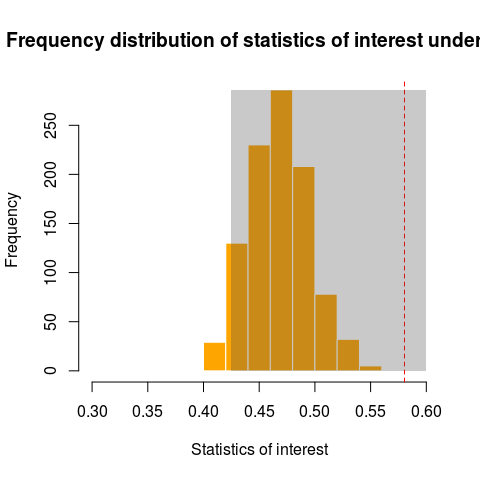Frequency distribution of the niche overlap index of aphids in 1000 simulations of the null hypothesis that the associations of aphids and plant species are independent. Zeroes (plants without the record of each species) were kept fixed. The red line shows the observed value of niche overlap.

Decision: do we reject the null hypothesis?

The observed value of our statistic of interest is within the acceptance region. Applying our significance criterion:

> sum(pielou.r1 <= pielou.si(pielou))/1000 < 0.05
 FALSE

Conclusion: we do not reject the null hypothesis. (p > 0,05).

1. Statistics.com LCC. 2009. Resampling Stats Add-in for Excel User’s Guide. http://www.resample.com/content/software/excel/userguide/RSXLHelp.pdf

2. There are simpler and faster ways to do this, but this one replicates the logic of drawing from an urn in Rsampling Stats